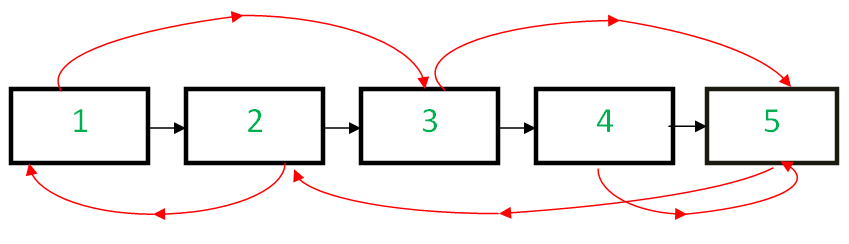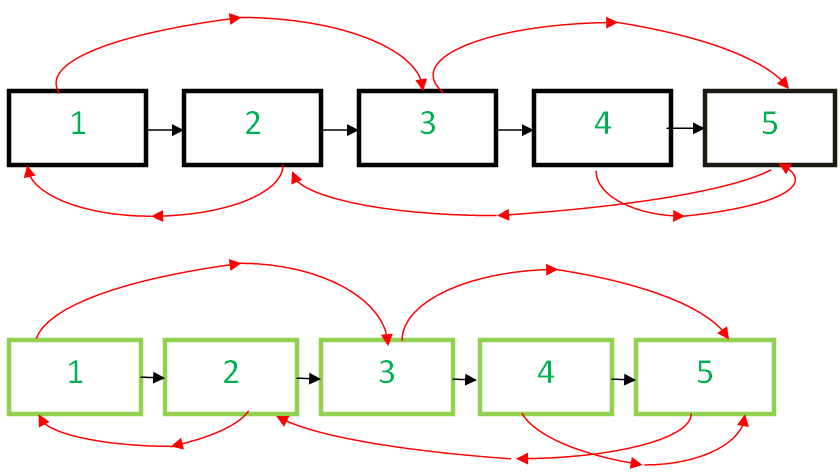# Javascript Program For Cloning A Linked List With Next And Random Pointer In O(1) Space

• Last Updated : 06 Jan, 2022

Given a linked list having two pointers in each node. The first one points to the next node of the list, however, the other pointer is random and can point to any node of the list. Write a program that clones the given list in O(1) space, i.e., without any extra space.
Examples:

`Input : Head of the below-linked list````Output :
A new linked list identical to the original list.```

In the previous posts Set-1 and Set-2 various methods are discussed, and O(n) space complexity implementation is also available.
In this post, we’ll be implementing an algorithm that’d require no additional space as discussed in Set-1.
Below is the Algorithm:

• Create the copy of node 1 and insert it between node 1 & node 2 in the original Linked List, create a copy of 2 and insert it between 2 & 3. Continue in this fashion, add the copy of N after the Nth node

• Now copy the random link in this fashion

``` original->next->random= original->random->next;  /*TRAVERSE
TWO NODES*/```
• This works because original->next is nothing but a copy of the original and Original->random->next is nothing but a copy of the random.

• Now restore the original and copy linked lists in this fashion in a single loop.

```original->next = original->next->next;
copy->next = copy->next->next;```
• Ensure that original->next is NULL and return the cloned listBelow is the implementation.

## Javascript

 ``
Output
```Original list :
Data = 1, Random  = 3
Data = 2, Random  = 1
Data = 3, Random  = 5
Data = 4, Random  = 5
Data = 5, Random  = 2

Cloned list :
Data = 1, Random  = 3
Data = 2, Random  = 1
Data = 3, Random  = 5
Data = 4, Random  = 5
Data = 5, Random  = 2
```

Please refer complete article on Clone a linked list with next and random pointer in O(1) space for more details!

My Personal Notes arrow_drop_up